# QlikView App Development

Discussion Board for collaboration related to QlikView App Development.

Highlighted
Contributor III

## How to sum sub level by dates ?

hi to all ,

i have projects , and in a project can be some books , each book has amount of unitss , and each project make savings of units.

i need to sum for a save-date of project the saved units until the save-date , and also the book-units that have any step until the save-date.

for example in this project for save-date 1/1/2016 there is 2 saved units , but no book units bcause any of the tree books has a step beffore 1/1/2016 .

for example in this project for save-date 1/6/2016 there is 15 saved units , and 104 book units bcause all of the tree books has a step beffore 1/6/2016 .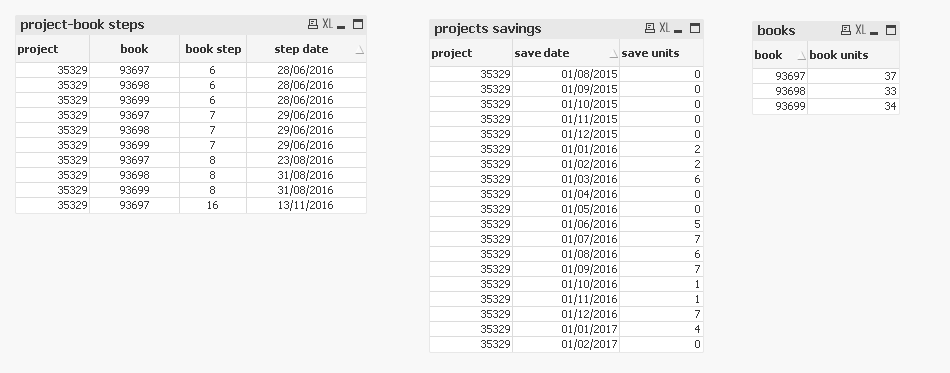i need to see project - save-date - saved units - book units  .

THANKS !

Tags (4)
1 Solution

Accepted SolutionsMVP

## Re: How to sum sub level by dates ?

May be this

Sum(Aggr(If([save date] >= Min([step date]), Sum([book units])), project, [save date], book))

15 RepliesMVP

## Re: How to sum sub level by dates ?

Would you be able to share a sample to play around with? and it would be helpful to know the numeric output you are looking to get here

Contributor III

## Re: How to sum sub level by dates ?

hi Sunny , here it is  ,

As i said ,

i need to see ( pivot )

dimension : project , save_date .

measures : sum of saved-units until save_date , sum of book-units than have any step until save_date.

THANKS!MVP

## Re: How to sum sub level by dates ?

Like I asked, I won't know what the numeric output you are looking to get.... If you are not sure what is the numeric output needed, then pick one example and explain the logic of what is needed for a single row so that we can deduce what is needed here

Contributor III

## Re: How to sum sub level by dates ?

hi Sunny ,  sorry for not being clear .  in this example you can see that only from the 1/8/2010 i have 3 saved units but no book from the 6 books had any step until this date . at the next record you can see that at the  1/9/2010 i have another 14 saved units so the          sum (save units) is 3+14=17 ,  And all the 6 books had a step at the 26/8/2010  so at the 1/9/2010 the sum (book units)  is 6 book * 6 units for each = 36.(- if there was only 2 books with steps in this date so it 2*6=12 )

at the next record you can see that at the  1/10/2010  there is no chage - no saved units , and all the books have steps from the prev month.

Note - sum (save units)  and  sum (book units)  are Aggregated values and only increase during time.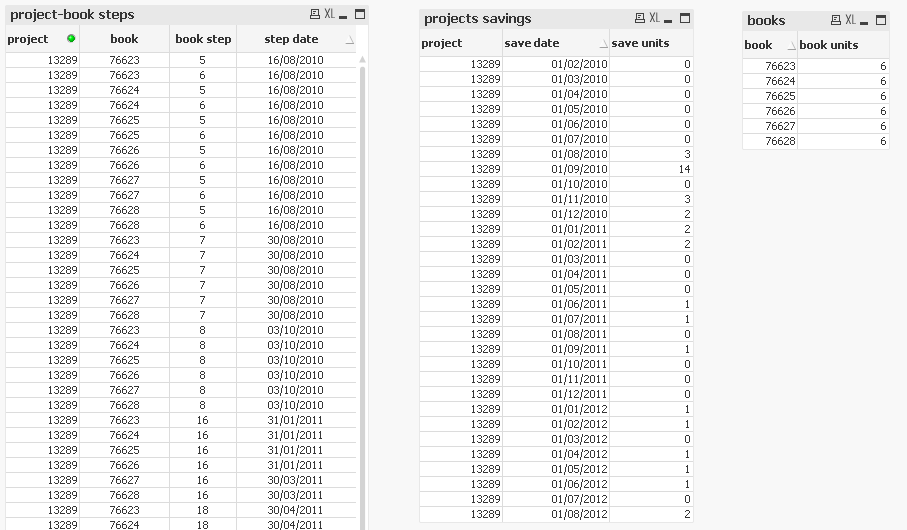A desired result -  ( pivot or any thing else .. )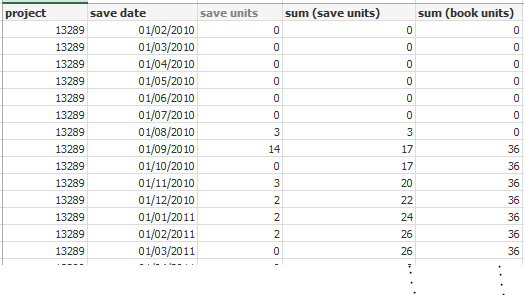hope its ok now .MVP

## Re: How to sum sub level by dates ?

Like this?Contributor III

## Re: How to sum sub level by dates ?

almost perfect ,

in this project there are no steps for any book at the 1/8/2010 or before , so sum (book units) have to be 0 at this date.

( sum (save units) is OK )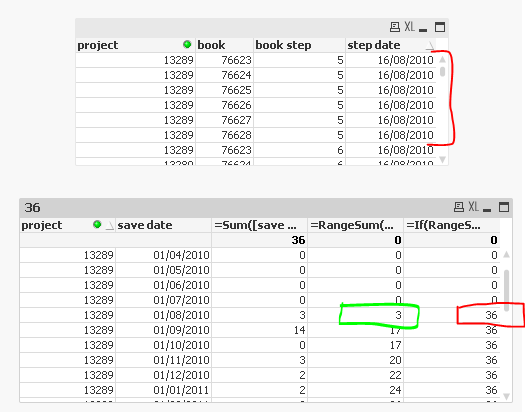MVP

## Re: How to sum sub level by dates ?

Check now... but check it with other selections to make sure that this is what you wanted

Contributor III

## Re: How to sum sub level by dates ?

sorry Sunny , i delete from source the first 2 steps for book 76623 so 6 units that belonge to it do not count until 3/10/2010 .

i need to see at the 1/9/2010  the value 30  for sum (book units) , but i see 36 .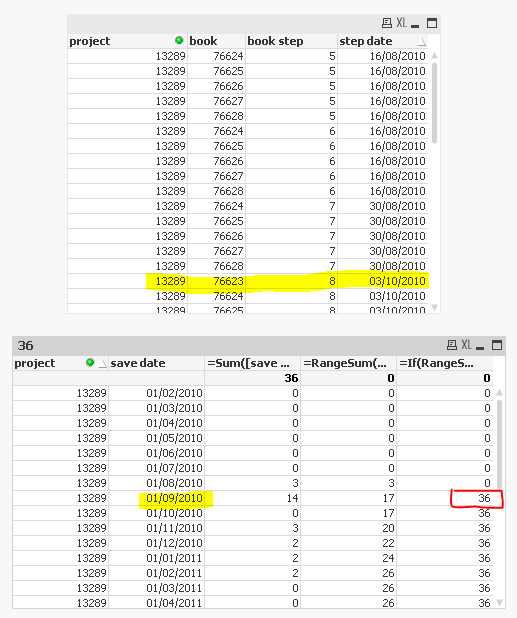BIG THANKS for trying to help me , I'm stuck ....MVP

## Re: How to sum sub level by dates ?

May be this

Sum(Aggr(If([save date] >= Min([step date]), Sum([book units])), project, [save date], book))